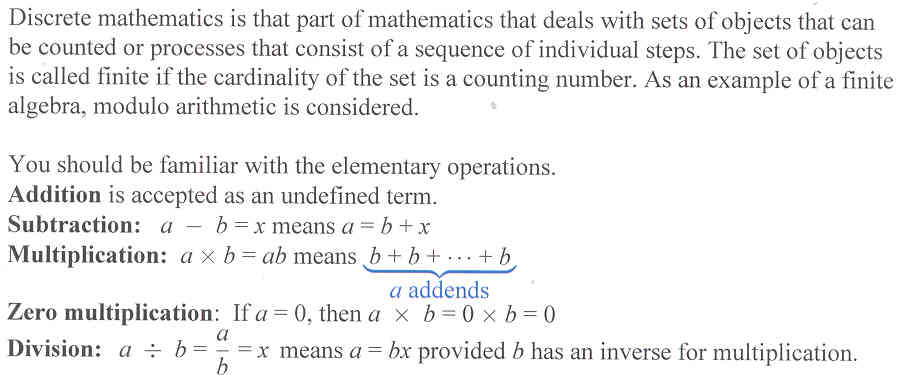# Section 5.7: Discrete Mathematics

## 5.7 Outline

1. Definition
2. Clock arithmetic
2. elementary operations
1. subtraction
2. multiplication
3. zero multiplication
4. division
3. Modulo five arithmetic
1. addition of a 5-hour clock
2. congruence modulo m
3. solving modular equations
4. Group properties for a modulo system
1. testing group properties
2. testing field properties

## 5.7 Essential Ideas